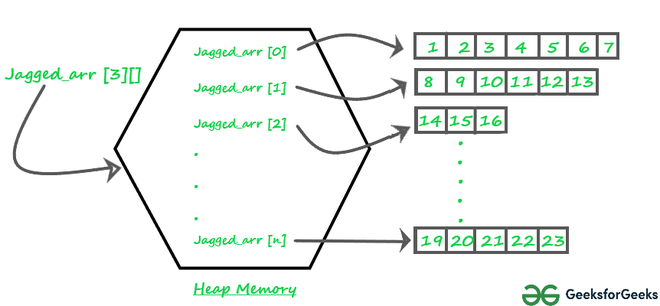Skip to content
Related Articles
Jagged Array in Java
• Difficulty Level : Easy
• Last Updated : 05 Feb, 2021

Prerequisite: Arrays in Java

A jagged array is an array of arrays such that member arrays can be of different sizes, i.e., we can create a 2-D array but with a variable number of columns in each row. These types of arrays are also known as Jagged arrays.

Pictorial representation of Jagged array in Memory:Jagged_array

Declaration and Initialization of Jagged array :

```Syntax: data_type array_name[][] = new data_type[n][];  //n: no. of rows
array_name[] = new data_type[n1] //n1= no. of colmuns in row-1
array_name[] = new data_type[n2] //n2= no. of colmuns in row-2
array_name[] = new data_type[n3] //n3= no. of colmuns in row-3
.
.
.
array_name[] = new data_type[nk]  //nk=no. of colmuns in row-n```

Alternative, ways to Initialize a Jagged array :

```                    int arr_name[][] = new int[][]  {
new int[] {10, 20, 30 ,40},
new int[] {50, 60, 70, 80, 90, 100},
new int[] {110, 120}
};

OR

int[][] arr_name = {
new int[] {10, 20, 30 ,40},
new int[] {50, 60, 70, 80, 90, 100},
new int[] {110, 120}
};

OR

int[][] arr_name = {
{10, 20, 30 ,40},
{50, 60, 70, 80, 90, 100},
{110, 120}
};```

Following are Java programs to demonstrate the above concept.

## Java

 `// Program to demonstrate 2-D jagged array in Java``class` `Main {``    ``public` `static` `void` `main(String[] args)``    ``{``        ``// Declaring 2-D array with 2 rows``        ``int` `arr[][] = ``new` `int``[``2``][];` `        ``// Making the above array Jagged` `        ``// First row has 3 columns``        ``arr[``0``] = ``new` `int``[``3``];` `        ``// Second row has 2 columns``        ``arr[``1``] = ``new` `int``[``2``];` `        ``// Initializing array``        ``int` `count = ``0``;``        ``for` `(``int` `i = ``0``; i < arr.length; i++)``            ``for` `(``int` `j = ``0``; j < arr[i].length; j++)``                ``arr[i][j] = count++;` `        ``// Displaying the values of 2D Jagged array``        ``System.out.println(``"Contents of 2D Jagged Array"``);``        ``for` `(``int` `i = ``0``; i < arr.length; i++) {``            ``for` `(``int` `j = ``0``; j < arr[i].length; j++)``                ``System.out.print(arr[i][j] + ``" "``);``            ``System.out.println();``        ``}``    ``}``}`
Output
```Contents of 2D Jagged Array
0 1 2
3 4
```

Following is another example where i’th row has i columns, i.e., the first row has 1 element, the second row has two elements and so on.

## Java

 `// Another Java program to demonstrate 2-D jagged``// array such that first row has 1 element, second``// row has two elements and so on.``class` `Main {``    ``public` `static` `void` `main(String[] args)``    ``{``        ``int` `r = ``5``;` `        ``// Declaring 2-D array with 5 rows``        ``int` `arr[][] = ``new` `int``[r][];` `        ``// Creating a 2D array such that first row``        ``// has 1 element, second row has two``        ``// elements and so on.``        ``for` `(``int` `i = ``0``; i < arr.length; i++)``            ``arr[i] = ``new` `int``[i + ``1``];` `        ``// Initializing array``        ``int` `count = ``0``;``        ``for` `(``int` `i = ``0``; i < arr.length; i++)``            ``for` `(``int` `j = ``0``; j < arr[i].length; j++)``                ``arr[i][j] = count++;` `        ``// Displaying the values of 2D Jagged array``        ``System.out.println(``"Contents of 2D Jagged Array"``);``        ``for` `(``int` `i = ``0``; i < arr.length; i++) {``            ``for` `(``int` `j = ``0``; j < arr[i].length; j++)``                ``System.out.print(arr[i][j] + ``" "``);``            ``System.out.println();``        ``}``    ``}``}`
Output
```Contents of 2D Jagged Array
0
1 2
3 4 5
6 7 8 9
10 11 12 13 14
```

This article is contributed by Rahul Agrawal. If you like GeeksforGeeks and would like to contribute, you can also write an article and mail your article to contribute@geeksforgeeks.org. See your article appearing on the GeeksforGeeks main page and help other Geeks.
Please write comments if you find anything incorrect, or you want to share more information about the topic discussed above

Attention reader! Don’t stop learning now. Get hold of all the important Java Foundation and Collections concepts with the Fundamentals of Java and Java Collections Course at a student-friendly price and become industry ready. To complete your preparation from learning a language to DS Algo and many more,  please refer Complete Interview Preparation Course.

My Personal Notes arrow_drop_up